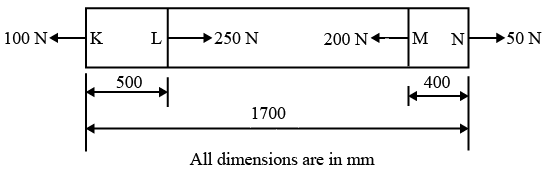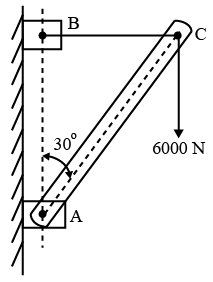# ISRO Scientist or Engineer Mechanical May 2017

Instructions

For the following questions answer them individually

Question 51

# What is the Prandtl number for a gas at $$27^\circ C$$ and $$1 \times 10^5$$ Pa, with with kinematic viscosity equal to $$1.8 \times 10^{-5} m^2/s$$, specific heat capacity at constant pressure = 700 J/kg-K and thermal conductivity of 0.02 W/m-K?(Take Gas constant = 300 J/kg-K)Question 52

# In continuous casting process, the mould is generally made ofQuestion 53

# A re-entry module flies with Mach 10 at an ambient temperature of $$-27^\circ C$$ with $$C_P$$ = 840 J/kg-K, gas constant R = 140 J/kg-K. What is the stagnation temperature at the tip of the nose of the re-entry module?Question 54

# Which one of the following steels contains least percentage of carbon?Question 55

# The Lewis number which is a measure of relative thermal and concentration boundary layer thicknesses is defined as, (Re: Reynold’s number, Pr: Prandtl number, Sc: Schmidt number)Question 56

# The figure shows an Aluminium rod of 25 mm$$^2$$ cross sectional area. It is loaded at four points, K, L, M and N. Assume E = 67 GPa for Aluminium. The total change in length of the rod due to loading as shown is close toQuestion 57

# The basis of slip line field theory in metal cutting isQuestion 58

# A room contains 25 kg. of air at 100 kPa and $$14^\circ C$$. The room has a 250 W refrigerator and 1 kW electric resistance heater. During a cold winter day, it is observed that both the refrigerator and the heater are running continuously, but the air temperature in the room remains constant. The rate of heat loss from the room during that day isQuestion 59

# The bar AC, 10 m long supports a load of 6000 N as shown in figure. The cable BC is horizontal and 5m long. Forces in the cable and the bar areQuestion 60

# In a grinding wheel specification of ‘wa36k8vbe’, “wa” indicatesOR